# GSEB Solutions Class 8 Maths Chapter 6 Square and Square Roots Ex 6.4

Gujarat Board GSEB Textbook Solutions Class 8 Maths Chapter 6 Square and Square Roots Ex 6.4 Textbook Questions and Answers.

## Gujarat Board Textbook Solutions Class 8 Maths Chapter 6 Square and Square Roots Ex 6.4

Question 1.
Find the square root of each of the following numbers by Division method?

1. 2304
2. 4489
3. 3481
4. 529
5. 3249
6. 1369
7. 5776
8. 7921
9. 576
10. 1024
11. 3136
12. 900

Solution:
1. We have:
∴ $$\sqrt{2304}$$ = 48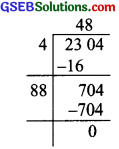2. We have:
∴ $$\sqrt{4489}$$ = 67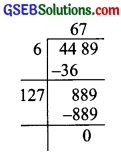3. We have:
∴ $$\sqrt{3481}$$ = 59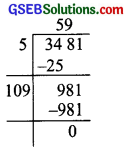4. We have:
∴ $$\sqrt{529}$$ = 23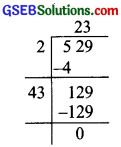5. We have:
∴ $$\sqrt{3249}$$ = 57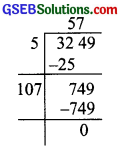6. We have:
∴ $$\sqrt{1369}$$ = 37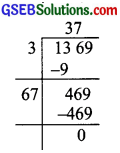7. We have:
∴ $$\sqrt{5776}$$ = 76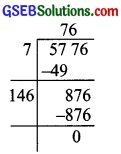8. We have:
∴ $$\sqrt{7921}$$ = 899. We have:
∴ $$\sqrt{576}$$ = 2410. We have:
∴ $$\sqrt{1024}$$ = 32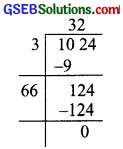11. We have:
∴ $$\sqrt{3136}$$ = 5612. We have:
∴ $$\sqrt{900}$$ = 30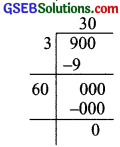Question 2.
Find the number of digits in the square root of each of the following numbers (without any calculation).

1. 64
2. 144
3. 4489
4. 27225
5. 390625

Solution:
If ‘V’ stands for number of digits in the given number, then

1. For 64, n = 2 [even number]
∴ Number of digit is its square root
= $$\frac{n}{2}$$ = $$\frac{2}{2}$$ = 1

2. For 144, n = 3 [odd number]
∴ Number of digits in its square root
= $$\frac{n+1}{2}$$ = $$\frac{3+1}{2}$$ = $$\frac{4}{2}$$ = 2

3. For 4489, n = 4 [even number]
Number of digits in its square root
= $$\frac{n}{2}$$ = $$\frac{4}{2}$$ = 2

4. For 27225, n = 5 [odd number]
∴ Number of digits in its square root
= $$\frac{n+1}{2}$$ = $$\frac{5+1}{2}$$
= $$\frac{6}{2}$$ = 3

5. For 390625, n = 6 [even number]
∴ Number of digits in its square root
= $$\frac{n}{2}$$ = $$\frac{6}{2}$$ = 3Question 3.
Find the square root of the following decimal numbers?

1. 2.56
2. 7.29
3. 51.84
4. 42.25
5. 31.36

Solution:
1. $$\sqrt{2.56}$$
Here, number of decimal places, are already even.
∴ We mark off’the periods and find the square root.
∴ $$\sqrt{2.56}$$ = 1.62. $$\sqrt{7.29}$$
Here, number of decimal places are already even. Therefore, we mark off the periods and find the square root.
∴ $$\sqrt{7.29}$$ = 2.7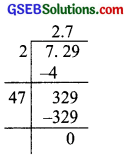3. $$\sqrt{51.84}$$
Here, the decimal places are already even.
∴ We mark off the periods and find the square root:
∴ $$\sqrt{51.84}$$ = 7.2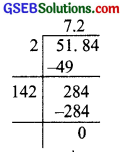4. $$\sqrt{42.25}$$
Here, the decimal places are already even.
∴ We mark off periods and find the square root:
∴ $$\sqrt{42.25}$$ = 6.5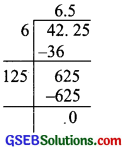5. $$\sqrt{31.36}$$
Here, the decimal places are already even.
∴ We mark off the periods and find the square root:
∴ $$\sqrt{31.36}$$ = 5.6Question 4.
Find the least number which must be subtracted from each of the following numbers so as to get a perfect square. Also find the square root of the perfect square so obtained?

1. 402
2. 1989
3. 3250
4. 825
5. 4000

Solution:
1. Since, we get a remainder 2
∴ The required least number to be subtracted from 402 is 2.
∴ 402 – 2 = 400, and $$\sqrt{400}$$ = 202. Since, we get a remainder of 53
∴ The least number to be subtracted from the given number = 53
1989 – 53 = 1936,
and $$\sqrt{1936}$$ = 44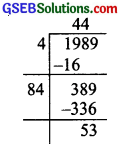3. Since, we get a remainder 1
∴ The smallest number to 53250 be subtracted from the given number = 1
Now, 3250 – 1 = 3249,
and $$\sqrt{3249}$$ = 574. Since, we get a remainder 41
∴ The required smallest number to be subtracted from the given number = 41
Now, 825 – 41 = 784,
and $$\sqrt{784}$$ = 28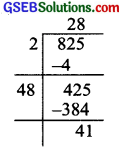5. Since, we get a remainder 31
∴ The required smallest number to be subtracted from the given number = 31
Now, 4000 – 31 = 3969,
and $$\sqrt{3969}$$ = 63Question 5.
Find the least number which must be added to each of the following numbers so as to get a perfect square. Also find the square root of the perfect square so obtained?

1. 525
2. 1750
3. 252
4. 1825
5. 6412

Solution:
1. Since, we get a remainder 41.
i.e; 525 > 222
and next square number is 23.
The required number to be added
= 232 – 525
= 529 – 525 = 4
Now, 524 + 4 = 529, and $$\sqrt{529}$$ = 23.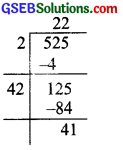2. Since, we get a remainder 69.
i.e., 1750 > (41)2
and next square number is 422.
∴ The required number to be added = 422 – 1750
= 1764 – 1750 = 14
Now, 1750 + 14 = 1764, and $$\sqrt{1764}$$
and $$\sqrt{1764}$$ = 42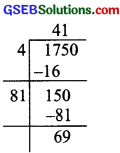3. Since, we get a remainder 27.
Since, 252 > (15)2 and next
square number = 16
∴ The required number to be added = 162 – 252
= 256 – 252 = 4
Now, 252 + 4 = 256, and $$\sqrt{256}$$ = 16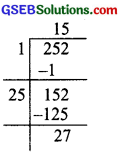4. Since, we get a remainder, 61.
∴ 1825 > (42)2
∵ Next square number = 43
∴ The required number to be added = (43)2 – 1825
= 1849 – 1825 = 24
Now, 1825 + 24 = 1849
and $$\sqrt{1849}$$ = 43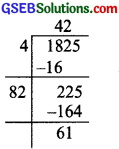5. Since, we get a remainder 12.
∴ 6412 > (80)2
∵ Next square number = 81
∴ Required number to be added = (81)2 – 6412
= 6561 – 6412 = 149
Now, 6412 + 149 = 6561 and $$\sqrt{6561}$$ = 81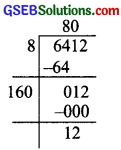Question 6.
Find the length of the side of a square whose area is 441 m2?
Solution:
Let the side of the square = x metre
∴ Area = side × side
= x × x = x2 metre2
∴ x2 = 441 ⇒ $$\sqrt{x^{2}}=\sqrt{441}$$
x = $$\sqrt{441}$$ = 21Thus, the required side is 21m.

Question 7.
In a right triangle ABC, ∠B = 90°.
(a) If AB = 6 cm, BC = 8 cm. find AC.
(b) If AC = 13 cm, BC = 5 cm, find AB.
Solution:
I. In a right triangle, the the hypotenuse.
II. (Hypotenuse)2 = [Sum of the squares of other two sides]
(a) ∵ ∠B = 90°
∴ Hypotenuse = AC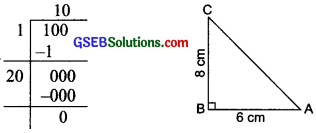∴ AC2 = AB2 + BC2 = 82 + 62 = 64 = 100
$$\sqrt{A C^{2}}$$ = $$\sqrt{100}$$
AC = 10
Thus, AC = 10 cm

(b) Here ∠B = 90°
∴ Hypotenuse = AC∵ AC2 = AB2 + BC2 or 132 = AB2 + 52
or AB2 = 132 – 52 = 169 – 25 = 144
Now $$\sqrt{A B^{2}}$$ = $$\sqrt{144}$$ or AB = 12
Thus, AB = 12 cm

Question 8.
A gardener has 1000 plants. He wants to plant these in such a way that the number of rows and the number of columns remain same. Find the minimum number of plants he needs more for this?
Solution:
Since, the number of plants in a row and the number of columns are the same.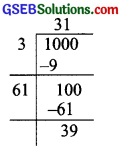∴ Their product must be a square number.
∵ The gardener has 1000 plants.
∴ 1000 is not a perfect square, and (31)2 < 1000
(∵ There is a remainder of 39).
Obviously the next square number = 32
∴ Number of plants required to be added
= (32)2 – 1000
= 1024 – 1000 = 24Question 9.
There are 500 children in a school. For a P.T. drill they have to stand in such a manner that the number of rows is equal to number of columns. How many children would be left out in this arrangement?
Solution:
Since, the number of rows and the number of columns are same.∴ Total number (i.e. their product) must be a square number, we have
Since, we get a remainder of 16
∴ 500 > (22)2 or 500 – 16 = (22)2
Thus, the required number of children to be left out = 16.